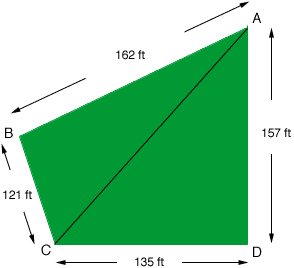Quandaries and Queries My name is Richard living in Las Vegas.  My wife and I are interested in buying property in Idaho but the owner can't give us a square footage of the lot.  The dimensions are as follows:   121.0 on the left side 157.0 on the right side 135.0 on the bottom 162.0 on the top   The bottom right corner of the lot is a true right angle, the rest are not. Hi Richard, The fact that the bottom left corner is a right angle makes it possible to calculate the area. I have drawn a rough sketch (not to scale) and labeled the corners so that I can make reference to them.The fact that angle CDA is a right angle means that the length of DA (157 ft) is the height of the triangle ACD and hence its area is  1/2baseheight = 1/2135157 = 10597.5 square feet The fact that angle CDA is a right angle also allows us to use Pythagoras Theorem to calculate the length of CA. By Pythagoras theorem |CA| = Sqrt(1352 + 1572) = 207.06 feet The final step is to calculate the area of the triangle ABC. This can be accomplished using Heron's Formula. I did this calculation and found the area of the triangle ABC to be 9788.4 square feet. Hence the area of your lot is 10597.5 + 9788.4 = 20,386 square feet. Penny Go to Math Central